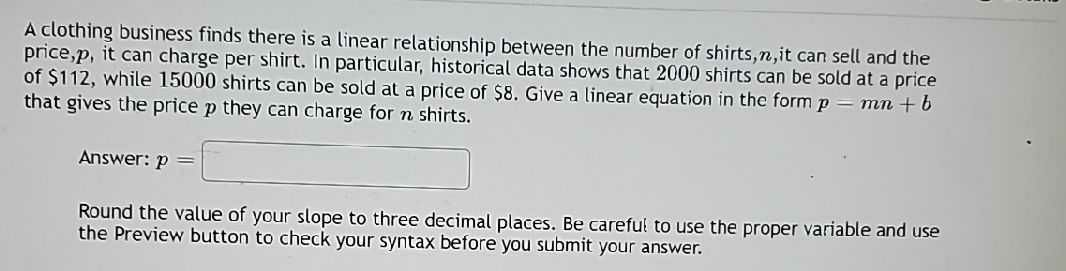### ¿Todavía tienes preguntas de matemáticas?

Pregunte a nuestros tutores expertos
Algebra
PreguntaA clothing business finds there is a linear relationship between the number of shirts, $$n$$ , it can sell and the price, $$p$$ , it can charge per shirt. In particular, historical data shows that $$2000$$ shirts can be sold at a price of $$\ 112$$ , while $$15000$$ shirts can be sold at a price of $$\ 8$$ . Give a linear equation in the form $$p - m n + b$$ that gives the price $$p$$ they can charge for $$n$$ shirts. Answer: $$p = \square$$ value of your slope to three decimal places. Be careful to use the proper variable and use Round the the Preview button to check your syntax before you submit your answer.

$$y= -0.008x+ 128$$